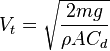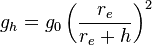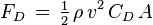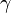## Monday, September 16, 2013

### An Interesting Question

The following question is one that I was wondering about recently:

At what height can the VIUD be dropped from and survive?

To answer this relatively simple question will take us down a variety of interesting paths which were not obvious at first.
To consider how our USB drive may survive we need to first look at failure mechanisms from dropping it from a considerable height:
• Impact -- Drive fails when hitting the ground.
• Temperature -- Drive fails due to heating from travelling at high velocity in air (like a meteor).

We'll first consider the impact failure mode since if the VIUD can't survive the ground impact we won't have to worry about temperature effects at higher speeds.

## Terminal Velocity

To consider whether our drive will survive impact with the ground when dropped from a given height we need to consider its terminal velocity. When an object is dropped in air it has two forces on it: the force of gravity down and the force of air in the opposite direction. The force of gravity on the object is essentially constant but as an object travels faster the the force of air resistance gets higher. At some point the force of air resistance equals the force of gravity and the object stops accelerating and reaches its terminal velocity.

The terminal velocity of an object is expressed in general as:where:
Vt = Terminal Velocity in m/s
m = Object's mass in kg
g = Force of gravity in m/s²
ρ = Density of air in kg/m³
A = Projected area of the object in the direction of motion in m²
Cd = Drag coefficient

Most of these parameters should be straight forward with the exception of the drag coefficient. Depending on the shape of the object its drag will be more or less which in turns affects its terminal velocity. We can use the measured coefficients of known shapes to estimate what it will be for our VIUD:
• Long Cylinder = 0.82 (direction parallel with axis)
• Short Cylinder = 1.15 (direction parallel with axis)
• Cylinder = 1.17 (direction perpendicular with axis)
• Sphere = 0.47
Depending on how our rugged drive falls it could have a drag coefficient anywhere from 0.82 to 1.17. Since the coefficient is inversely proportional to the terminal velocity the smallest coefficient will result in the largest, or worst case, terminal velocity so we'll use Cd = 0.82 for the rest of the calculations.

Plugging in all our values (assuming worst case in everything):

m = 0.06 kg
g = 9.8 m/s²
ρ = 1.22 kg/m³
A =  0.00041 m²
Cd = 0.82
Vt = 54 m/s (195 km/hr, 121 mile/hr)

From this estimation we know that no matter how high we drop our USB drive it should hit the ground at a maximum of 54 m/s.

## Terminal Velocity as a Function of Height

Our previous calculation was only interested in the VIUD's terminal velocity at ground level but for the next step we will need to know its terminal velocity at any given height above the ground. Since the density of air drops as we go higher the estimated terminal velocity will increase as higher altitudes.

We first need to find the density of air as a function of height. We can use an analytic equation for the density of air within the troposphere, or roughly up to 15 km in height and there are also a variety of more complex air density calculations if you look for them. What we'll end up using is simply a table of measured air densities which also conveniently has the force of gravity which does decrease slightly within the first 100 km. More detailed tables are also available if you need finer altitude steps.

Using this air density table and the same VIUD parameters as before yields the following graph of the terminal velocity versus altitude:

We can assume for simplicity that above around 100 km the air density is so small that there is no effective terminal velocity.

## Velocity of a Dropped Object as a Function of Height

The next step in the modelling is to figure out what the velocity profile of an object dropped at a specific height is. Assuming the object is dropped far from the surface of the earth there will be three basic areas to consider:
1. Space - No terminal velocity.
2. Upper Atmosphere - Terminal velocity begins to drop rapidly.
3. Lower Atmosphere - Object reaches a steady velocity of 54 m/s.

To determine acceleration in space we simply need to know the force due to gravity from Earth (we'll ignore all other astronomical bodies for simplicity):$g_h=g_0\left(\frac{r_e}{r_e+h}\right)^2$

where:
go = 9.8 m/s2
re = Mean radius of earth, 6371 km
h = Altitude above the mean radius of Earth in km

While the object is in space computing its velocity is relatively straightforward. The fact that the force of gravity increases as its approaches Earth makes it more complex but it can be solved with a simple numerical integration at regular intervals from the drop height to 100 km where the terminal velocity begins to take effect.

To figure out the net acceleration of the object once it hits the atmosphere we'll need to know the drag force which is where the prior terminal velocity equation was derived from:$F_D\, =\, \tfrac12\, \rho\, v^2\, C_D\, A$

Our net force on the falling object within the atmosphere is then expressed as:

Fnet = mgh - Fd

Since both the force of gravity and terminal velocity depends on height we'll keep doing a numerical integration solution. Graphing the velocity versus altitude for a couple of different drop heights yields the following velocity profiles:

Assuming we did everything correct in the solver the graphs appear to make sense. As the object falls in space it continuously picks up speed until it hits the atmosphere at around 80-100 km. From there its velocity begins to quickly decline as it hits more dense air. At around 10 km it reaches its final terminal velocity of 54 m/s falling at roughly a constant speed.

We can do a quick check to make sure our model is outputting reasonable numbers. If we use the equation for the velocity of a falling object after a given distance, sqrt(2gd), and take the force of gravity at the midpoint of the 10,000 km drop height (3.1 m/s2) we find a velocity of 7700 m/s at 100 km which is reasonably close to the model's value of 8500 m/s.

## Aerodynamic Heating

We're finally at a point we can find what we're looking for: the temperature of the object as it falls through the atmosphere at a high velocity, otherwise known as aerodynamic heating. While our VIUD is a relatively simple cylindrical shape it is still too complex for a simple modelling of aerodynamic heating. Realistically we would want to do some finite element modelling but this is beyond my current capabilities so we'll have to settle for some approximations.

All the simple aerodynamic heating models I've seen have assumed a thin plate travelling with the thin edge towards the direction of motion. This isn't too far from our VIUD but since it is thicker than a plate we could assume the heating would be more severe than that of a plate (how severe is difficult to guess).

where:

Twad = Temperature of air just beside object, K
T = Temperature of air far from object, K
r = Recovery factor, estimate for air of 0.84-0.87
M = Speed of object in Machs$\gamma$ = Heat capacity ratio, 1.40

We can get the normal air temperature as a function of altitude from the same data tables we used for air density. The heat capacity ratio doesn't change far from a value of 1.4 for air until you get into temperatures above 1000oC which is irrelevant for this model.

From this presentation we'll use the very simple approximation to find the temperature of the USB body:

Tnet = Tair + 0.5 (Tobj - Tair) + 0.22 (Twad - Tair)

I'm unsure exactly how they derived this but I'm assuming it is only valid for a particular model and may not be accurate at all for our case. Unfortunately, all other methods I've investigated are extremely complex and a little beyond my limited understanding of heat dynamics (we'll look at alternate temperature models in another post). Since this model depends on temperature and speed of the object we'll do a numerical integration just like for our velocity calculation which yields a graph like:

Not surprisingly there are large temperature increases when the fast moving object first begins to hit the atmosphere. While it is difficult to tell from this graph the actual amount of time spent at these temperature is relatively small: for temperatures above 1000 K only 10 seconds are spent for the object dropped at 10000 km and 19 seconds for the object dropped at 1000 km. The object dropped from further up experiences a higher temperature spike but for less time as it is moving much faster (8 km/s compared to 4 km/s).

Since the melting point of Aluminum is only 933 K (660 °C, 1220 °F) we can guess that the objects dropped above 1000 km would have significant damage, assuming they survived at all. The object dropped at 100 km experiences a much gentler temperature curve only reaching a maximum of 410 K (137 °C, 280 °F) and only being above the boiling point of water for 10 seconds. This is far below what our USB drive has survived in temperature testing so we can be reasonably sure of its survival in this case.

Although, if we were to be optimistic for the higher altitude drops, at the high altitudes the air density is very low (a million times lower than that at sea level) and the amount time experienced at high temperatures so low (temperature rating for the VIUD at 2300 K  for 40 seconds) that a more accurate temperature model could reveal the VIUD can survive drops from higher altitudes.

## Conclusion (Kind Of)

Although the accuracy of our temperature results is a little suspect and unverified we have at least a reasonable guess that dropping our rugged USB drive from up to 100 km will not destroy it from temperature affects. More research into an accurate temperature and heat model needs to be done to get more accurate results, assuming we want them.